# Scientific Communication CITS 7200 Lecture 3 The Inclusion

• Slides: 63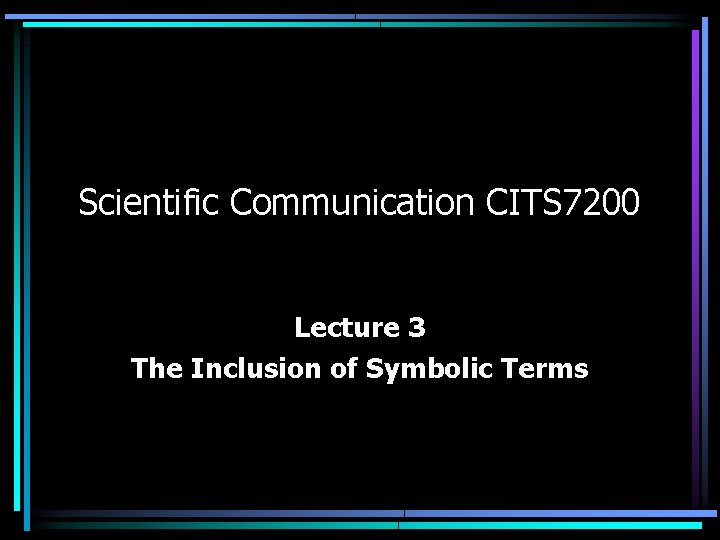Scientific Communication CITS 7200 Lecture 3 The Inclusion of Symbolic TermsDo not start a sentence with a symbol. • n(t) is a small noise component. • A small noise component is denoted by n(t).• • src_pid is a parameter passed by reference. The parameter src_pid is passed by reference.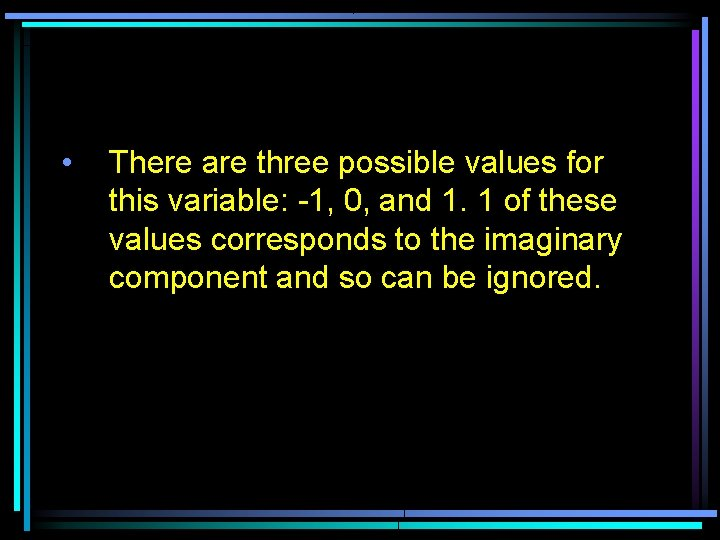• There are three possible values for this variable: -1, 0, and 1. 1 of these values corresponds to the imaginary component and so can be ignored.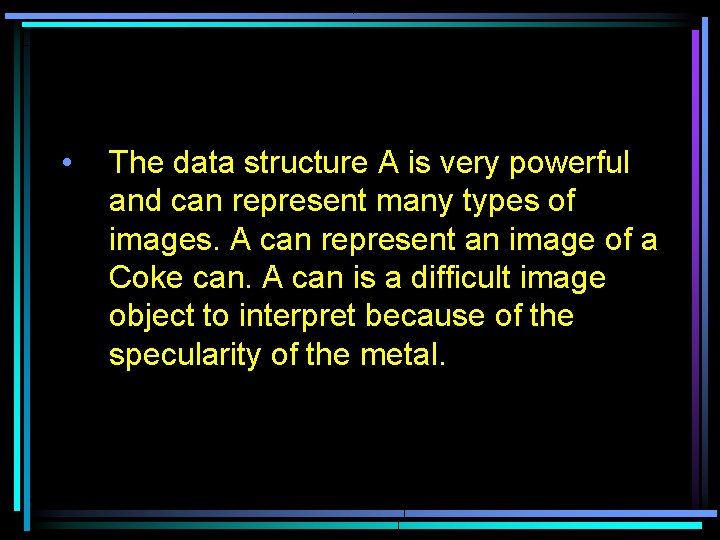• The data structure A is very powerful and can represent many types of images. A can represent an image of a Coke can. A can is a difficult image object to interpret because of the specularity of the metal.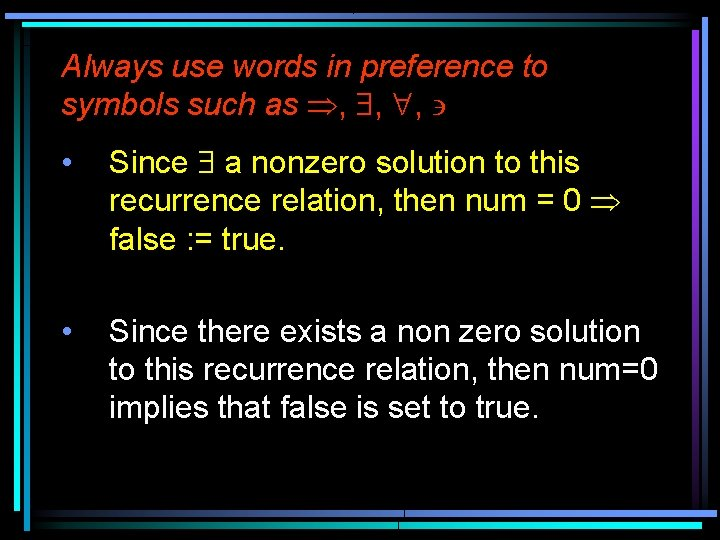Always use words in preference to symbols such as Þ, \$, ", ' • Since \$ a nonzero solution to this recurrence relation, then num = 0 Þ false : = true. • Since there exists a non zero solution to this recurrence relation, then num=0 implies that false is set to true.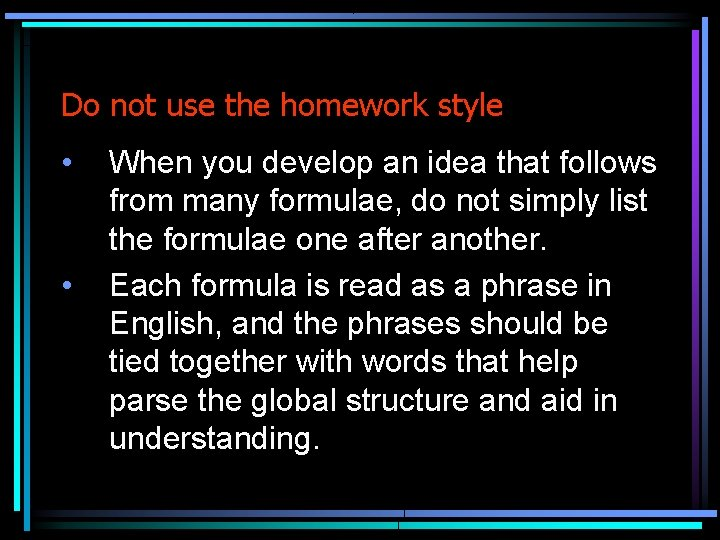Do not use the homework style • • When you develop an idea that follows from many formulae, do not simply list the formulae one after another. Each formula is read as a phrase in English, and the phrases should be tied together with words that help parse the global structure and aid in understanding.Avoid subscripts and superscripts • Let X = {x 1, . . . , xn} and consider a subset of X, say S = {xi 1, . . . , xim}. • Let X be a finite set and consider a subset S of X. Let x be a member of S.• Do not write Xi in one place, and then Xn in another • Do not call a vector (x 1, x 2, x 3) in one place, and then (x, y, z) in another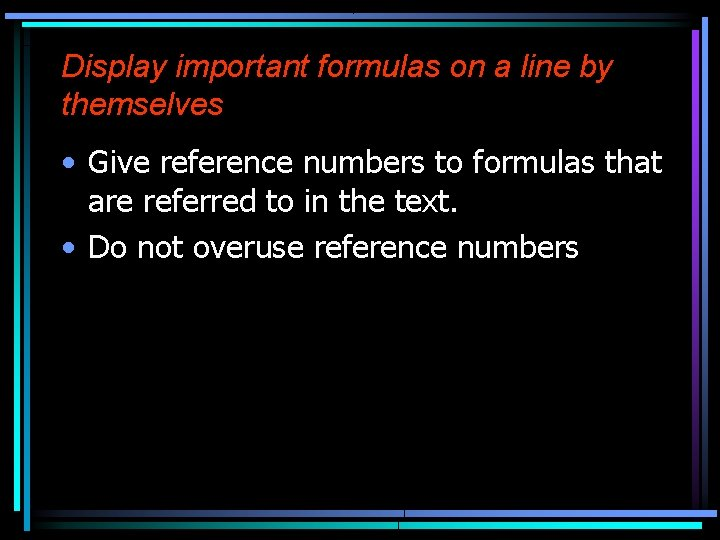Display important formulas on a line by themselves • Give reference numbers to formulas that are referred to in the text. • Do not overuse reference numbers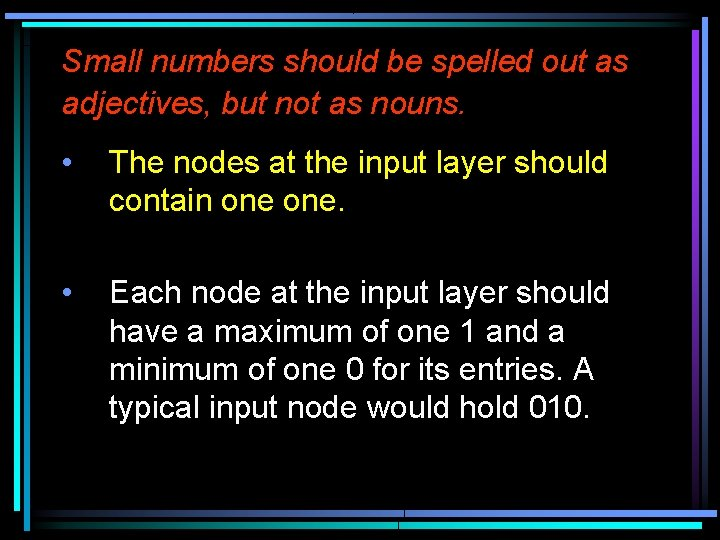Small numbers should be spelled out as adjectives, but not as nouns. • The nodes at the input layer should contain one. • Each node at the input layer should have a maximum of one 1 and a minimum of one 0 for its entries. A typical input node would hold 010.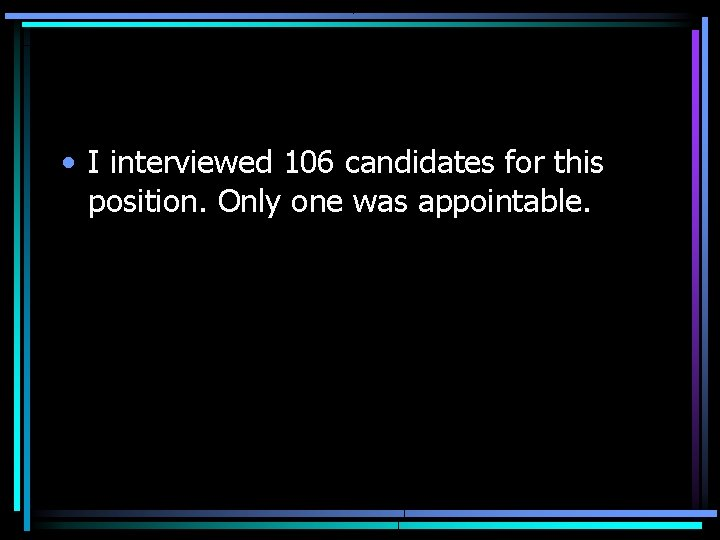• I interviewed 106 candidates for this position. Only one was appointable.• The method requires 2 passes. • Method 3 is illustrated in Figure 1; it requires two passes.Do not mix modes There were between four and 32 processors in each machine. There were between 4 and 32 processors in each machine.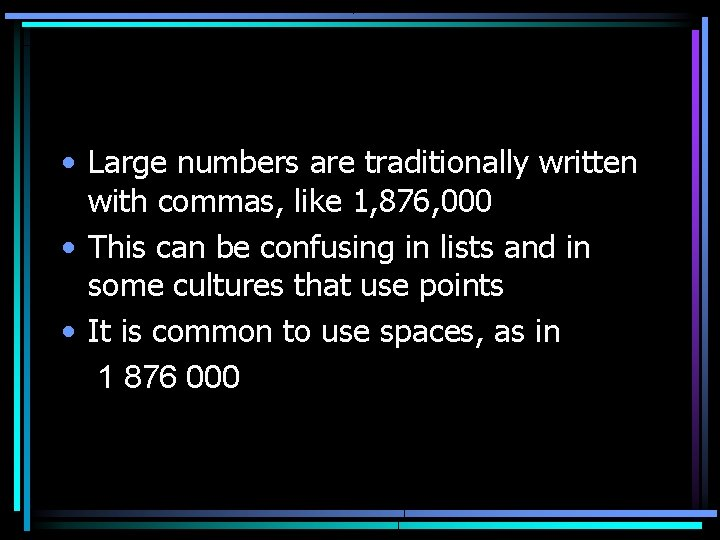• Large numbers are traditionally written with commas, like 1, 876, 000 • This can be confusing in lists and in some cultures that use points • It is common to use spaces, as in 1 876 000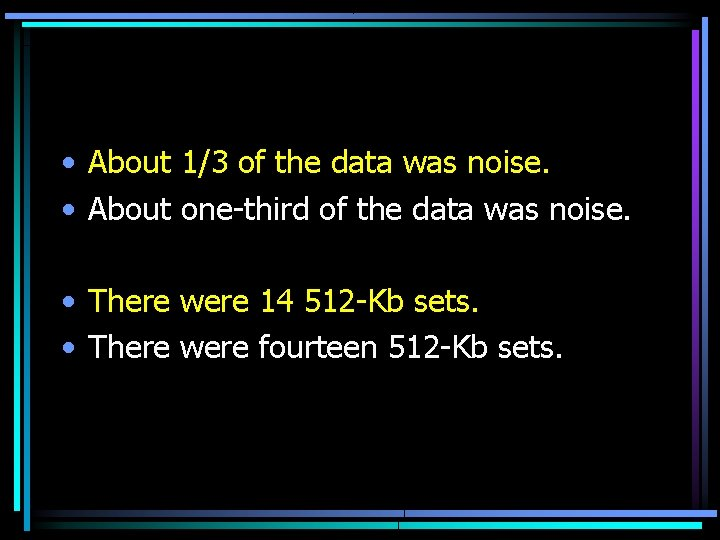• About 1/3 of the data was noise. • About one-third of the data was noise. • There were 14 512 -Kb sets. • There were fourteen 512 -Kb sets.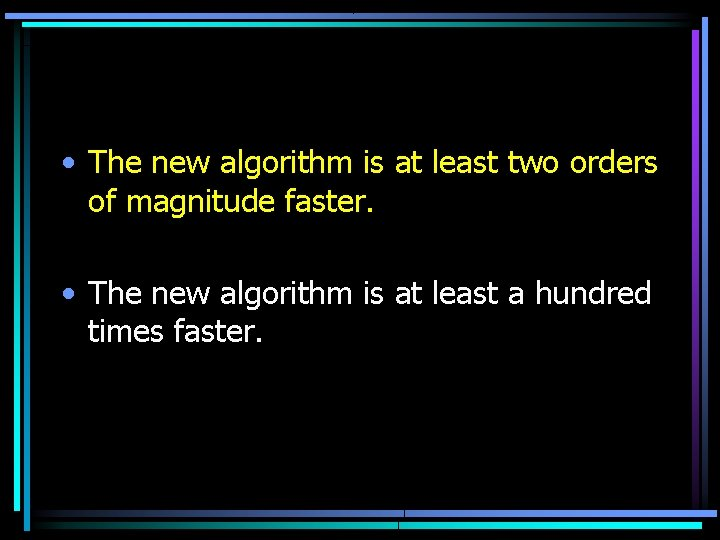• The new algorithm is at least two orders of magnitude faster. • The new algorithm is at least a hundred times faster.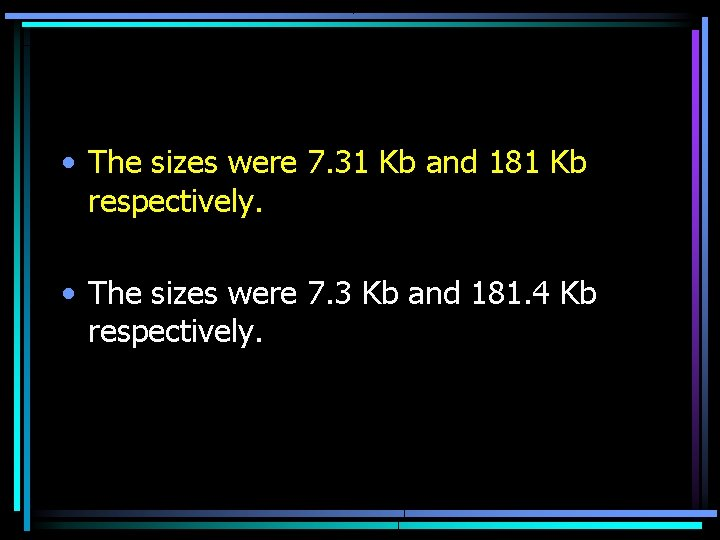• The sizes were 7. 31 Kb and 181 Kb respectively. • The sizes were 7. 3 Kb and 181. 4 Kb respectively.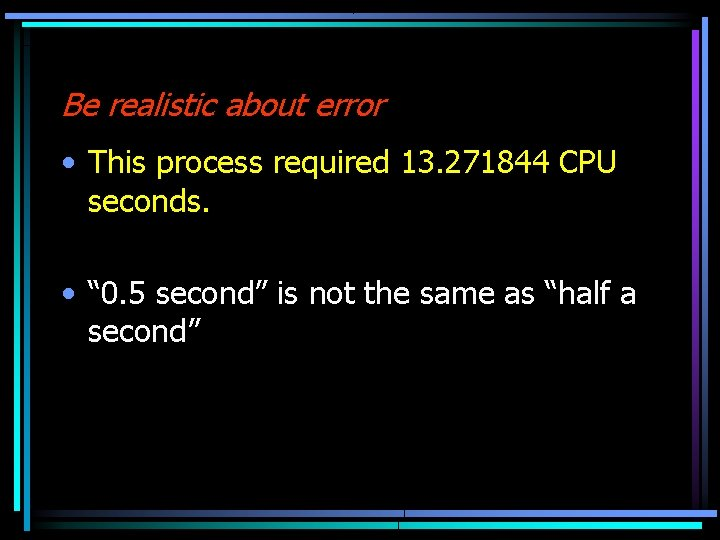Be realistic about error • This process required 13. 271844 CPU seconds. • “ 0. 5 second” is not the same as “half a second”Units of measurement • Space and time common • Time units are the second (sec), minute (min) and hour (hr) • It is unusual to give abbreviated forms • ms (millisecond) might be thought as microsecond (ms) • Use colons 3: 30 minutes• Basic units of space are bit and byte • These are combined in tenth power of 2 rather than third power of 10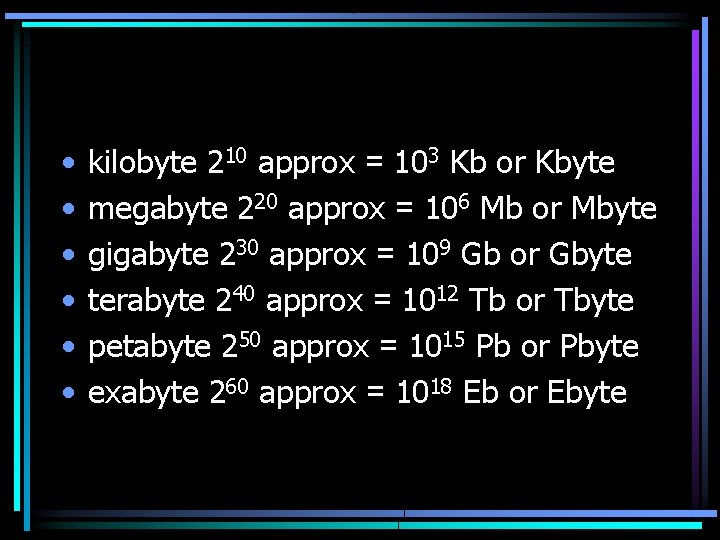• • • kilobyte 210 approx = 103 Kb or Kbyte megabyte 220 approx = 106 Mb or Mbyte gigabyte 230 approx = 109 Gb or Gbyte terabyte 240 approx = 1012 Tb or Tbyte petabyte 250 approx = 1015 Pb or Pbyte exabyte 260 approx = 1018 Eb or Ebyte• Choose units that are easy to understand • Does 1. 50 minutes mean one and a half minutes or one minute and 50 seconds? • Some units are not well defined, MIPS• For quantities greater than 1, the unit is plural; less than 1 and it’s singular • The average run took 1. 3 seconds, and the fastest took 0. 8 second.Do not use superfluous mathematical symbols • This algorithm has t = log 2 n loops in the worst case.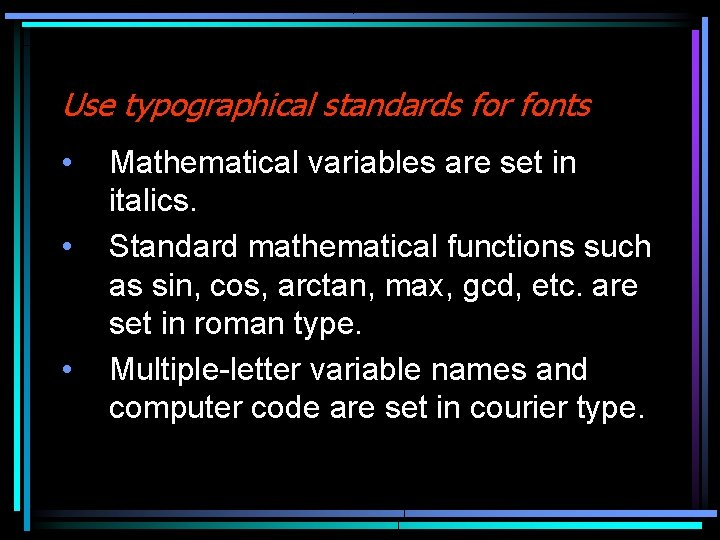Use typographical standards for fonts • • • Mathematical variables are set in italics. Standard mathematical functions such as sin, cos, arctan, max, gcd, etc. are set in roman type. Multiple-letter variable names and computer code are set in courier type.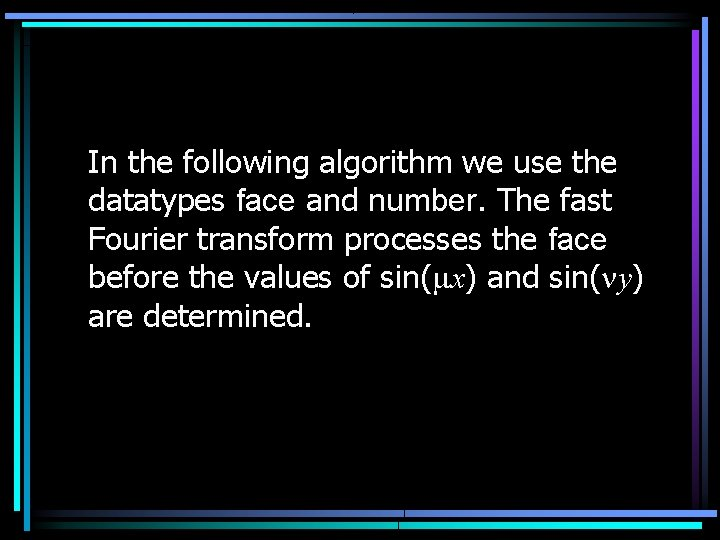In the following algorithm we use the datatypes face and number. The fast Fourier transform processes the face before the values of sin(mx) and sin(ny) are determined.• The symbols e and d denote small quantities • i, j, k, m and n are integers (although i and j also represent imaginary units) • The symbols p and e are fundamental constants.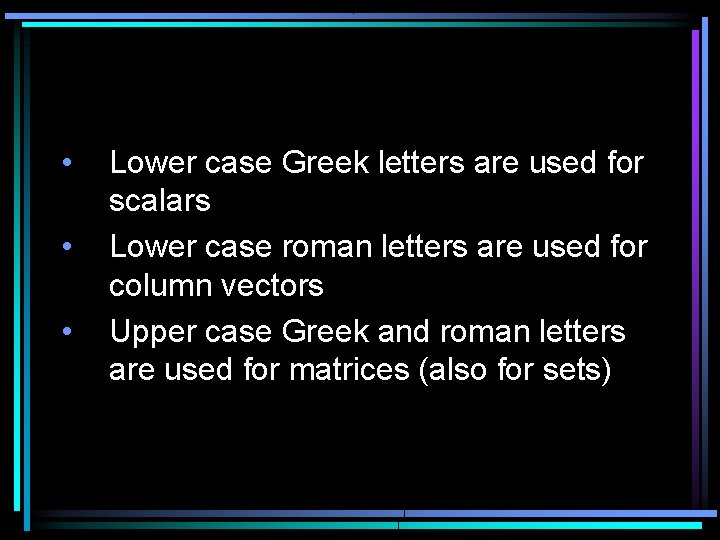• • • Lower case Greek letters are used for scalars Lower case roman letters are used for column vectors Upper case Greek and roman letters are used for matrices (also for sets)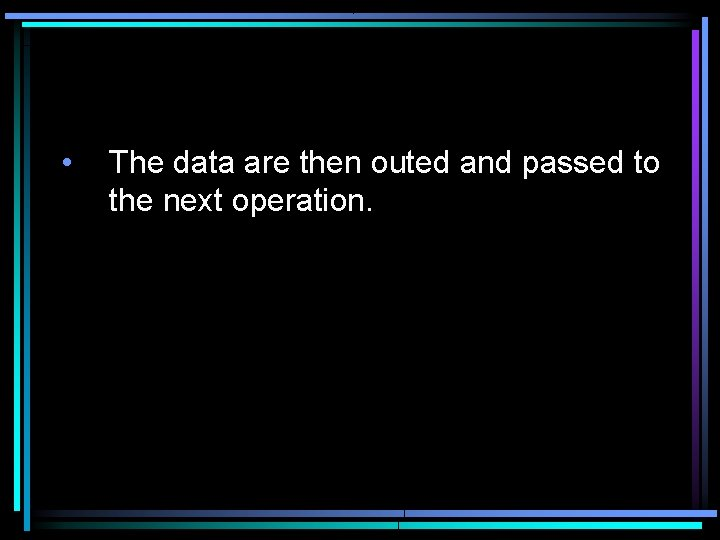• The data are then outed and passed to the next operation.Symbols in distinct formulas must be separated by words. • Let P be a set of n points Mi, i = 1, . . . , n of ¶X. • Let P be a set of n points Mi, where i = 1, . . . , n and each Mi is a member of ¶X.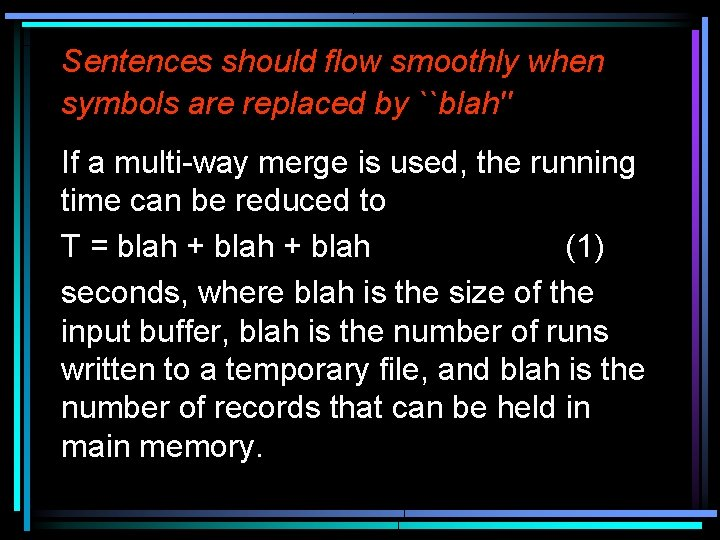Sentences should flow smoothly when symbols are replaced by ``blah'' If a multi-way merge is used, the running time can be reduced to T = blah + blah (1) seconds, where blah is the size of the input buffer, blah is the number of runs written to a temporary file, and blah is the number of records that can be held in main memory.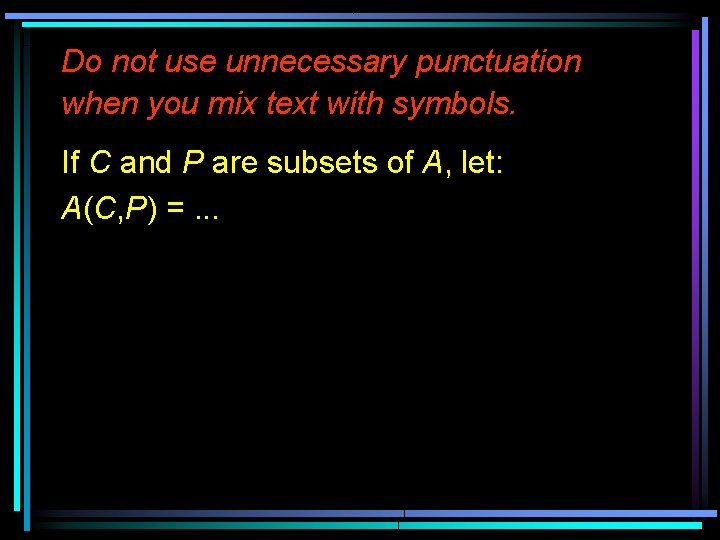Do not use unnecessary punctuation when you mix text with symbols. If C and P are subsets of A, let: A(C, P) =. . .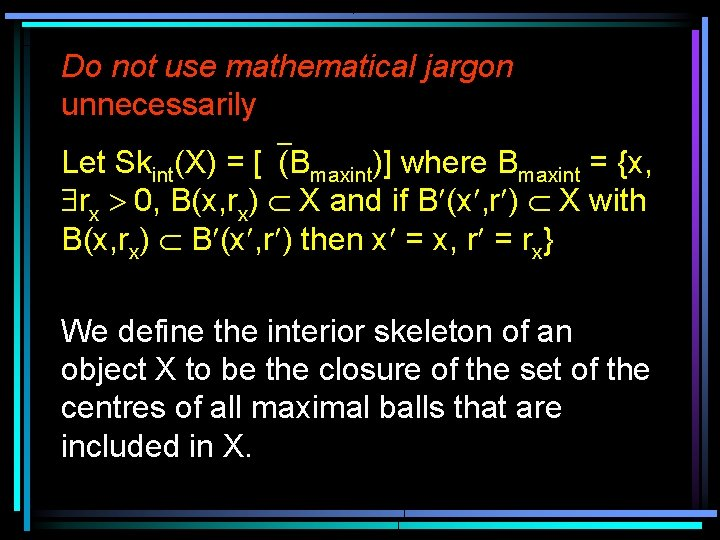Do not use mathematical jargon unnecessarily Let Skint(X) = [`(Bmaxint)] where Bmaxint = {x, \$rx > 0, B(x, rx) Ì X and if B¢(x¢, r¢) Ì X with B(x, rx) Ì B¢(x¢, r¢) then x¢ = x, r¢ = rx} We define the interior skeleton of an object X to be the closure of the set of the centres of all maximal balls that are included in X.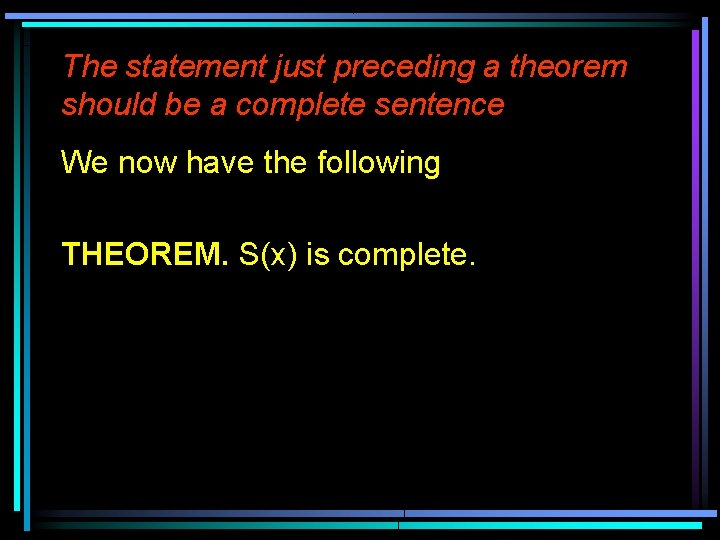The statement just preceding a theorem should be a complete sentence We now have the following THEOREM. S(x) is complete.We can now prove the following result. THEOREM. The state space function S(x) defined in (1. 5) is complete.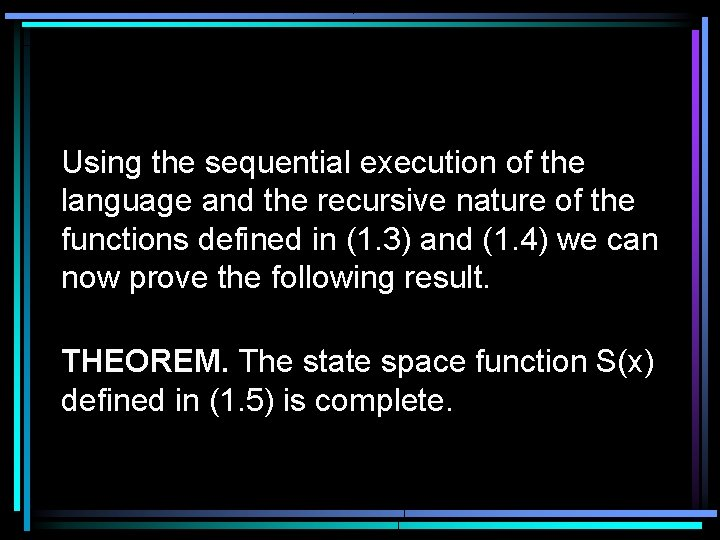Using the sequential execution of the language and the recursive nature of the functions defined in (1. 3) and (1. 4) we can now prove the following result. THEOREM. The state space function S(x) defined in (1. 5) is complete.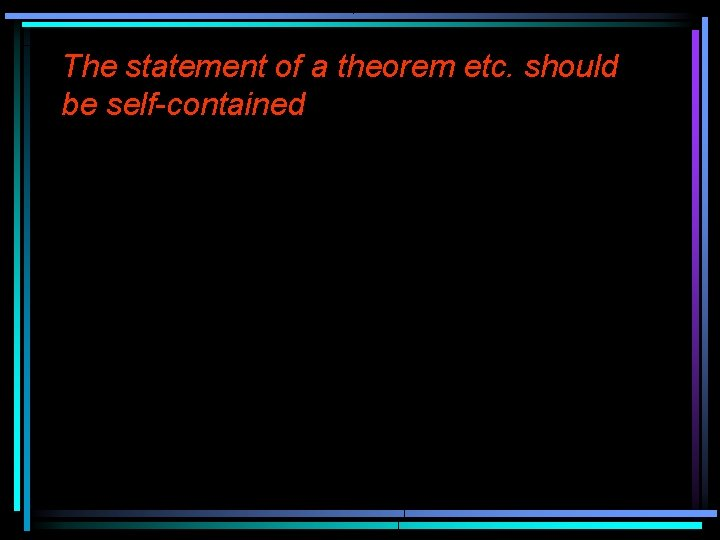The statement of a theorem etc. should be self-contained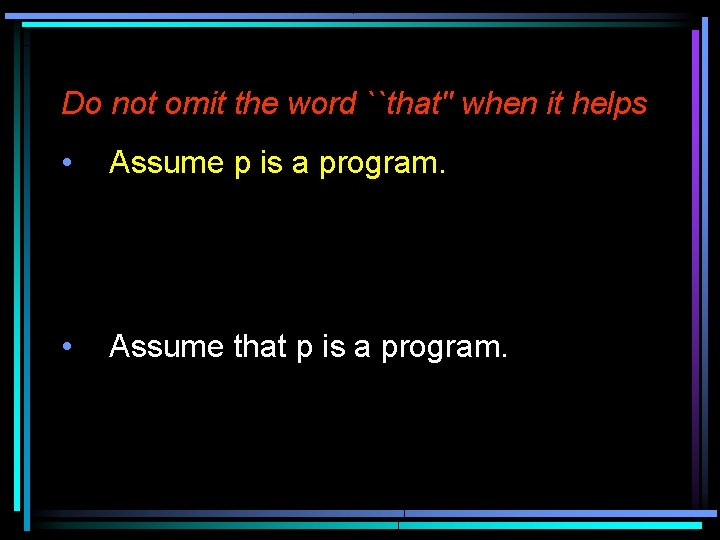Do not omit the word ``that'' when it helps • Assume p is a program. • Assume that p is a program.• … we have that … • … because of the fact that …Parallelism • Whilst we imagine that Euclidean geometry best describes the world, in fact vision and graphics are better defined in terms of perspective geometry.• Whilst we imagine that Euclidean geometry best describes the world, perspective geometry is more appropriate for vision and graphics.Formerly, science was taught by the textbook method, while now the laboratory method is employed. Formerly, science was taught by the textbook method; now it is taught by the laboratory method.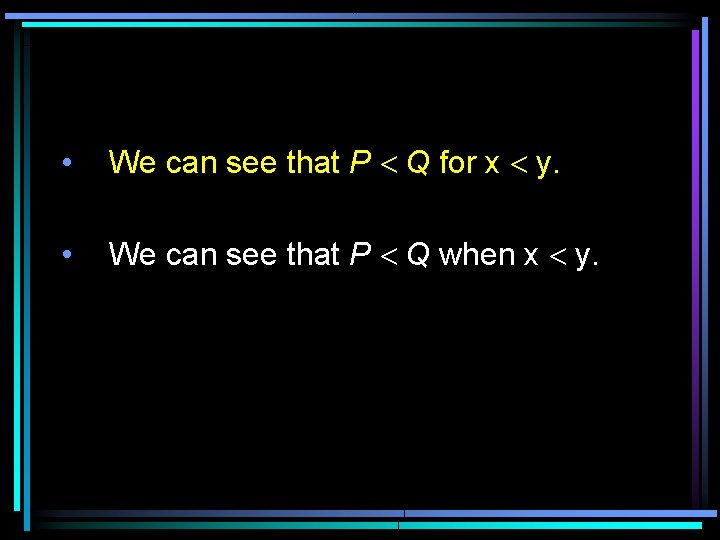• We can see that P < Q for x < y. • We can see that P < Q when x < y.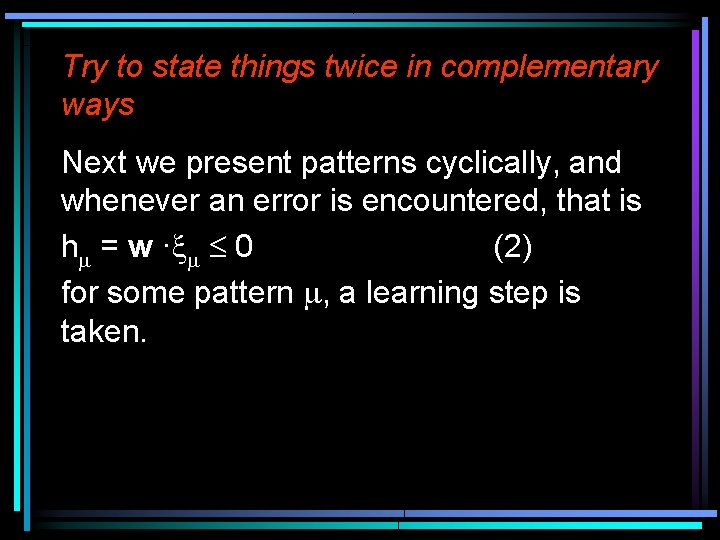Try to state things twice in complementary ways Next we present patterns cyclically, and whenever an error is encountered, that is hm = w ·xm £ 0 (2) for some pattern m, a learning step is taken.Motivate the reader for what follows. • We now prove that our algorithm terminates after a finite number of steps.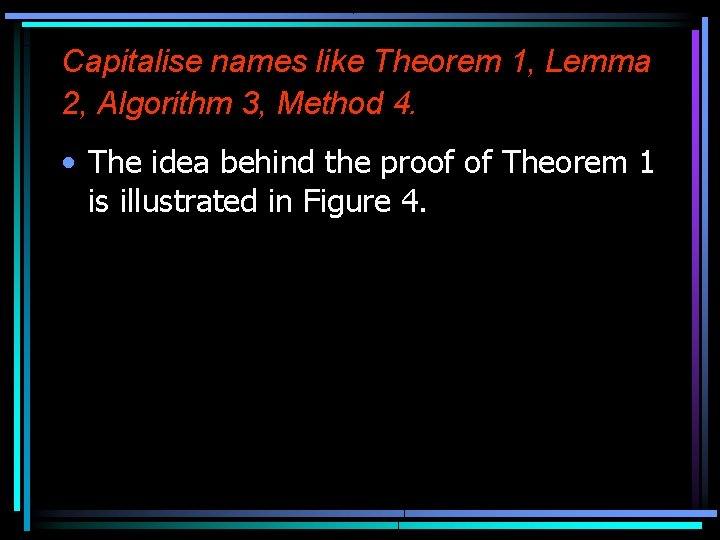Capitalise names like Theorem 1, Lemma 2, Algorithm 3, Method 4. • The idea behind the proof of Theorem 1 is illustrated in Figure 4.Use a spelling checker! complement not compliment She paid him an enormous compliment when she showed him how to complement his code with comments.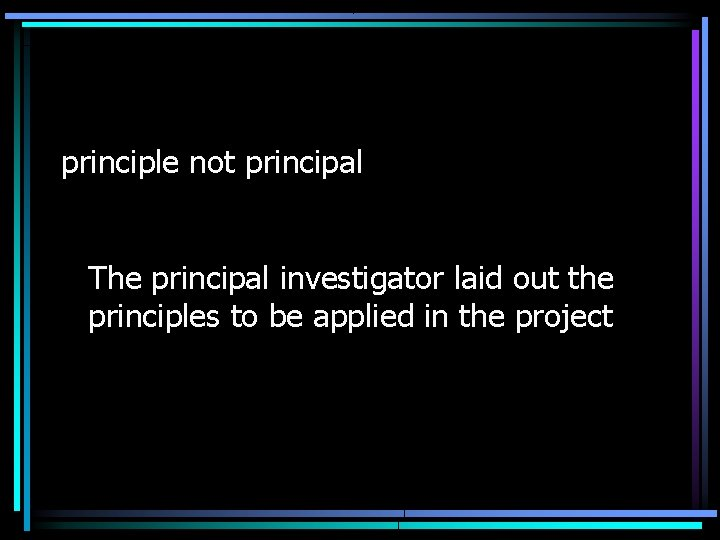principle not principal The principal investigator laid out the principles to be applied in the project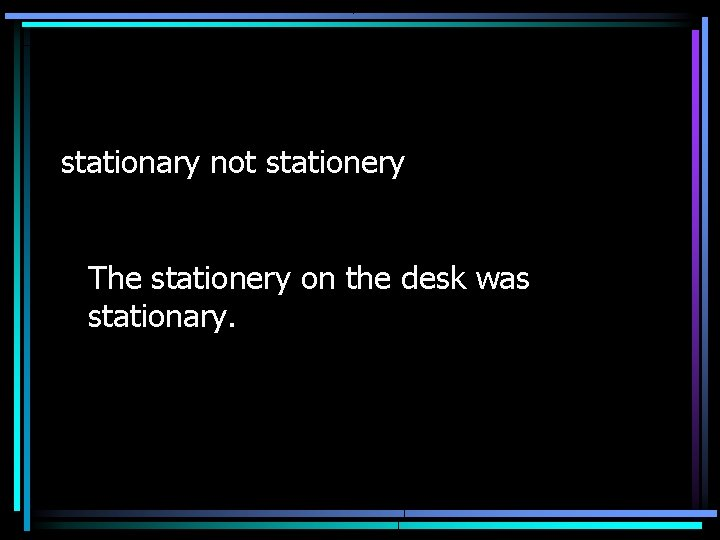stationary not stationery The stationery on the desk was stationary.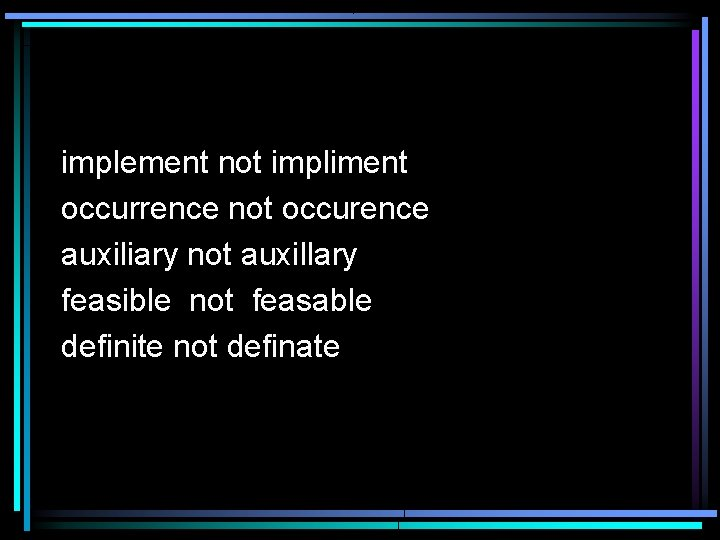implement not impliment occurrence not occurence auxiliary not auxillary feasible not feasable definite not definate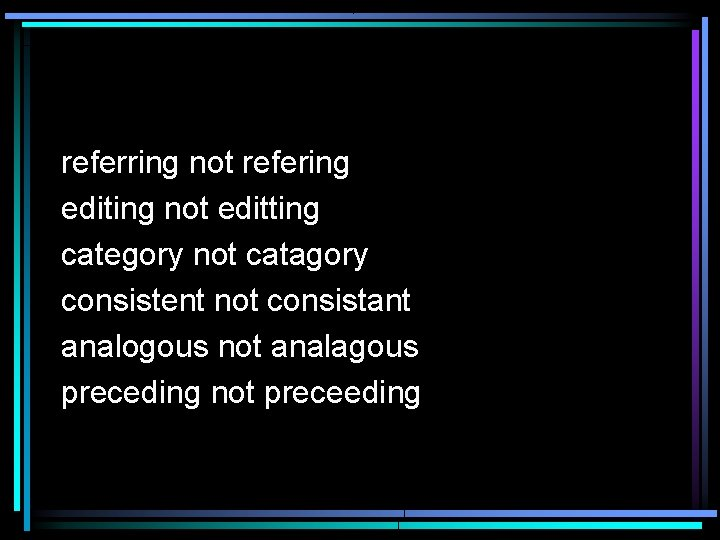referring not refering editing not editting category not catagory consistent not consistant analogous not analagous preceding not preceeding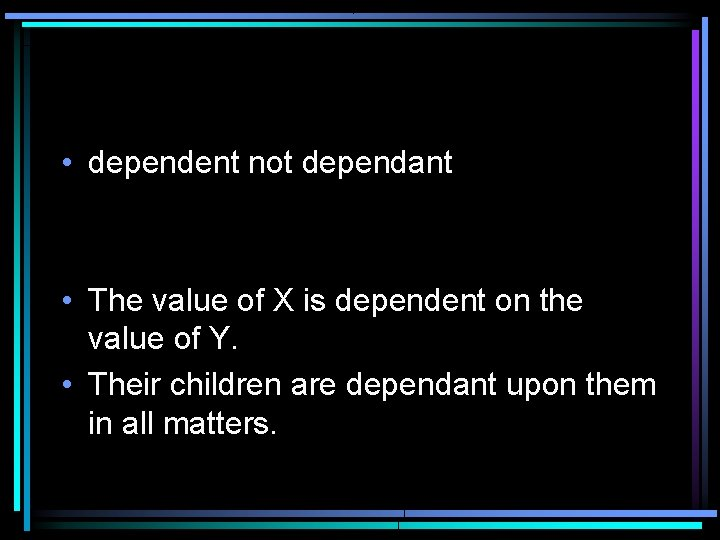• dependent not dependant • The value of X is dependent on the value of Y. • Their children are dependant upon them in all matters.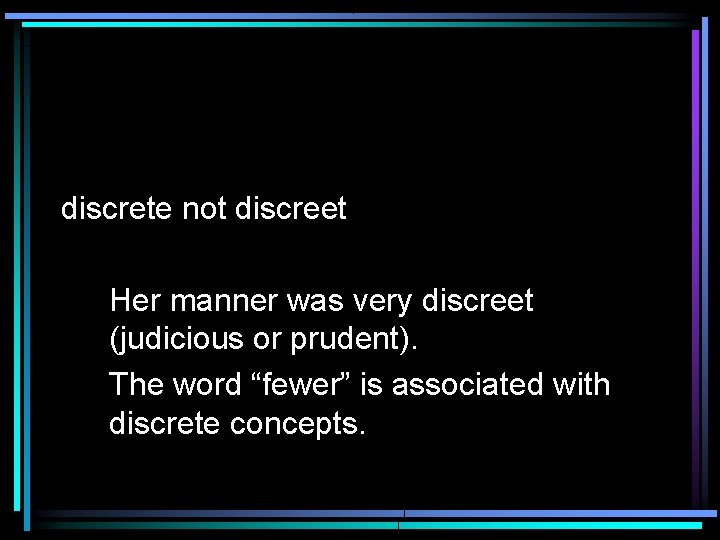discrete not discreet Her manner was very discreet (judicious or prudent). The word “fewer” is associated with discrete concepts.affect (verb) not effect (noun) The effect of this variable is huge. It affects all other variables in the method.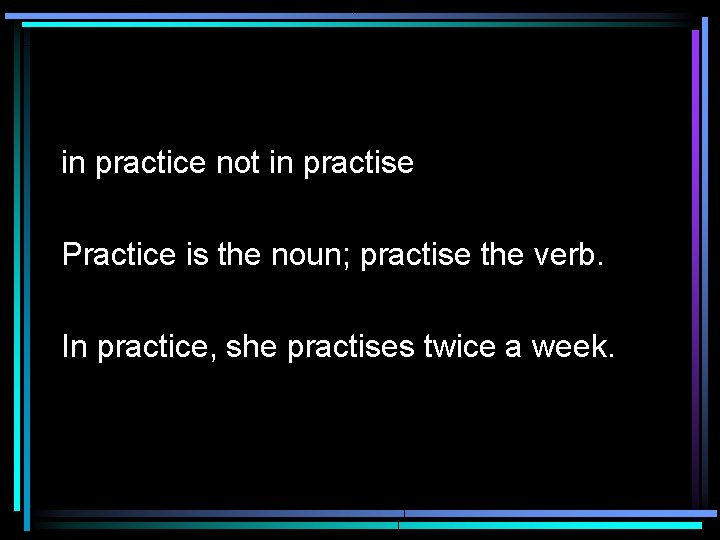in practice not in practise Practice is the noun; practise the verb. In practice, she practises twice a week.• descendant not descendent • While both spellings have existed in the past, the spelling is now always –ant.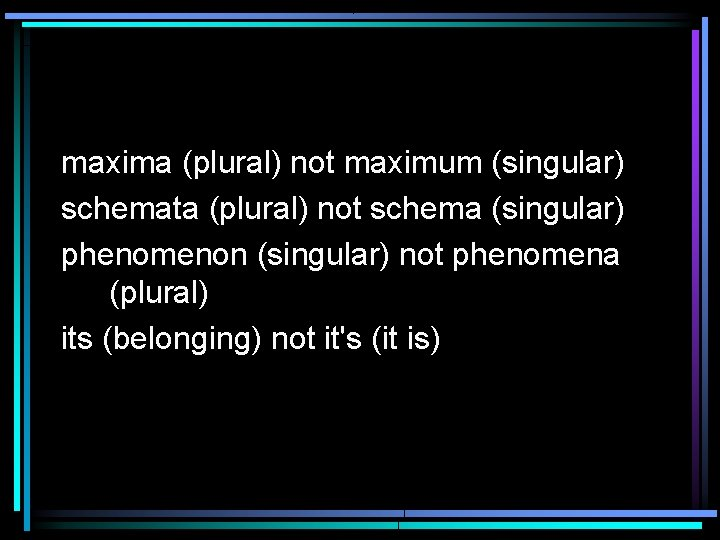maxima (plural) not maximum (singular) schemata (plural) not schema (singular) phenomenon (singular) not phenomena (plural) its (belonging) not it's (it is)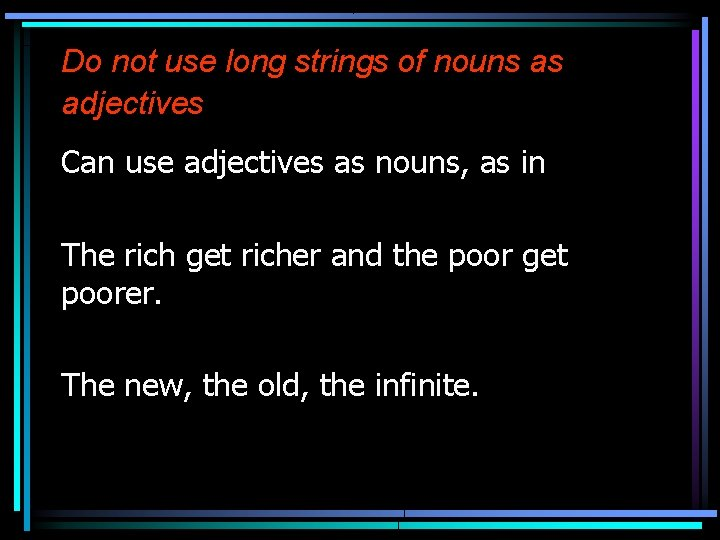Do not use long strings of nouns as adjectives Can use adjectives as nouns, as in The rich get richer and the poor get poorer. The new, the old, the infinite.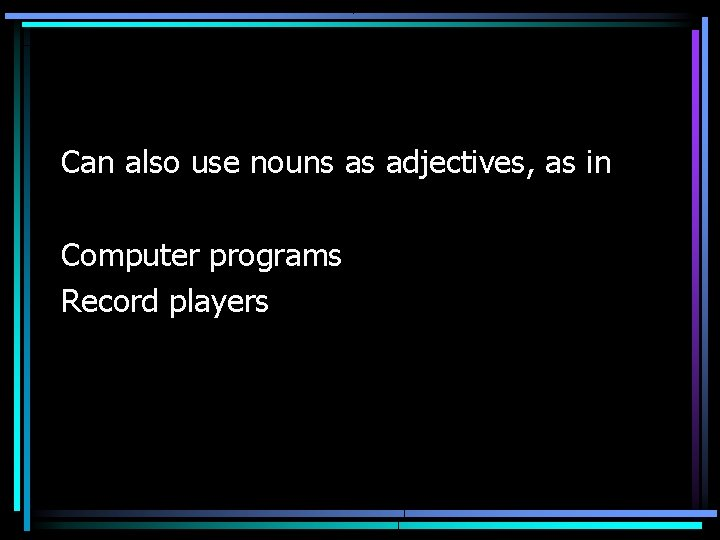Can also use nouns as adjectives, as in Computer programs Record players• Too many adjectives undermine the argument. • Frowning angrily, she moved hurriedly towards him, saying very harshly, “You bastard. ” • Scowling, she stalked towards him. “You bastard. ”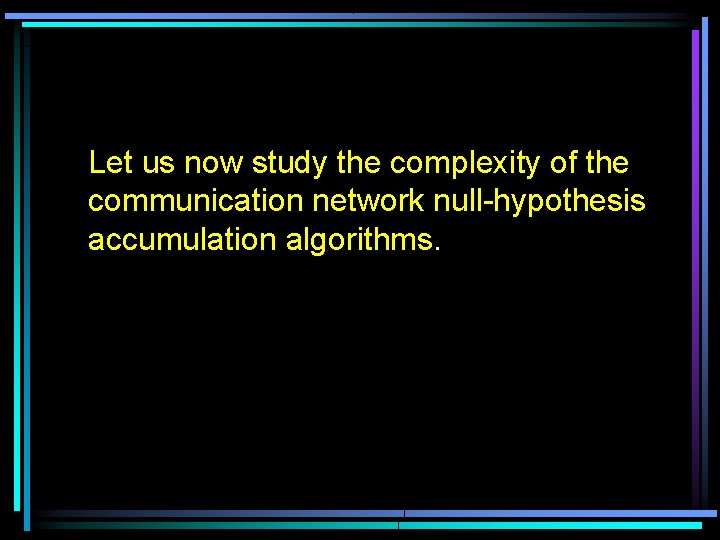Let us now study the complexity of the communication network null-hypothesis accumulation algorithms.